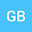Ellipses and hyperbolas of decompositions of even numbers into pairs of prime numbers
•## Abstract

This is just an attempt to associate sums or dierences of prime numbers with points lying on an ellipse or hyperbola. Certain pairs of prime numbers can be represented as radius-distances from the focuses to points lying either on the ellipse or on the hyperbola.
The ellipse equation can be written in the following form: |p k | + |p t | = 2n.
The hyperbola equation can be written in the following form: ||p k | − |p t || = 2n.
Here p k and p t are prime numbers (p 1 = 2, p 2 = 3, p 3 = 5, p 4 = 7,...), k and t are indices of prime numbers, 2n is a given even number, k, t, n ∈ N.
If we construct ellipses and hyperbolas based on the above, we get the following:
1) there are only 5 non-intersecting curves (for 2n=4; 2n=6; 2n=8; 2n=10; 2n=16). The remaining ellipses have intersection points;
2) there is only 1 non-intersecting hyperbola (for 2n=2) and 1 non-intersecting vertical line. The remaining hyperbolas have intersection points.